中华流行病学杂志2017, Vol. 38Issue (3): 400-405PDF
http://dx.doi.org/10.3760/cma.j.issn.0254-6450.2017.03.025

0

#### 文章信息

Gao Wenlong, Lin He, Liu Xiaoning, Ren Xiaowei, Li Juansheng, Shen Xiping, Zhu Suling.

Evaluation of estimation of prevalence ratio using bayesian log-binomial regression model

Chinese journal of Epidemiology, 2017, 38(3): 400-405
http://dx.doi.org/10.3760/cma.j.issn.0254-6450.2017.03.025

### 文章历史

1. 730000 兰州大学公共卫生学院流行病与卫生统计研究所;
2. 730000 兰州大学信息与工程学院计算机软件与理论研究所

Evaluation of estimation of prevalence ratio using bayesian log-binomial regression model
Gao Wenlong1, Lin He2, Liu Xiaoning1, Ren Xiaowei1, Li Juansheng1, Shen Xiping1, Zhu Suling1
1. Department of Epidemiology and Health Statistics, School of Public Health, Lanzhou University, Lanzhou 730000, China;
2. Department of Computer Software, School of Information and Engineering, Lanzhou University, Lanzhou, 730000, China
Corresponding author: Gao Wenlong, Email: gaowl06@aliyun.com
Fund program: Humanities and Social Sciences Foundation of the Ministry of Education of China (15XJC910001); Fundamental Research Funds for the Universities Subordinated to Central Government of China (lzujbky-2016-025)
Abstract: To evaluate the estimation of prevalence ratio (PR) by using bayesian log-binomial regression model and its application, we estimated the PR of medical care-seeking prevalence to caregivers' recognition of risk signs of diarrhea in their infants by using bayesian log-binomial regression model in Openbugs software. The results showed that caregivers' recognition of infant's risk signs of diarrhea was associated significantly with a 13% increase of medical care-seeking. Meanwhile, we compared the differences in PR's point estimation and its interval estimation of medical care-seeking prevalence to caregivers' recognition of risk signs of diarrhea and convergence of three models (model 1:not adjusting for the covariates; model 2:adjusting for duration of caregivers' education, model 3:adjusting for distance between village and township and child month-age based on model 2) between bayesian log-binomial regression model and conventional log-binomial regression model. The results showed that all three bayesian log-binomial regression models were convergence and the estimated PRs were 1.130(95%CI:1.005-1.265), 1.128(95%CI:1.001-1.264) and 1.132(95%CI:1.004-1.267), respectively. Conventional log-binomial regression model 1 and model 2 were convergence and their PRs were 1.130(95%CI:1.055-1.206) and 1.126(95%CI:1.051-1.203), respectively, but the model 3 was misconvergence, so COPY method was used to estimate PR, which was 1.125 (95%CI:1.051-1.200). In addition, the point estimation and interval estimation of PRs from three bayesian log-binomial regression models differed slightly from those of PRs from conventional log-binomial regression model, but they had a good consistency in estimating PR. Therefore, bayesian log-binomial regression model can effectively estimate PR with less misconvergence and have more advantages in application compared with conventional log-binomial regression model.
Key words: Bayes theorem     Regression analysis     Models, statistical     Prevalence ratio     Log-binomial regression

log-binomial回归模型：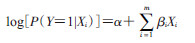(1)

Deggens等提出了扩充原始数据集，在扩充的数据集上运行log-binomial回归模型能够获得最大似然的近似估计值。扩充原始数据集的步骤：当log-binomial回归模型不收敛时，将原始数据集中Y＝1的个案增加c-1倍，然后，再将原始数据集中Y值互换，将这两个新的数据集合并成一个数据集，即为复制（COPY）数据集。再利用COPY数据集拟合log-binomial回归模型从而达到解决模型不收敛问题。通常c为常数，c越大，COPY数据集的伪似然估计值越接近于最大似然估计值。在SAS软件中，可以利用genmod模块中的加权log-binomial回归模型实现COPY数据集下的log-binomial回归方法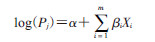(2)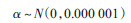(3)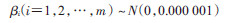(4)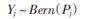(5)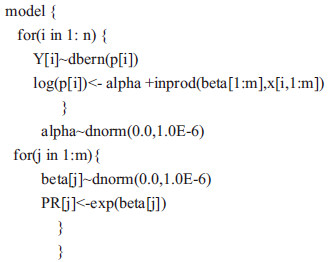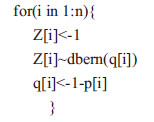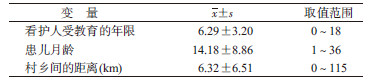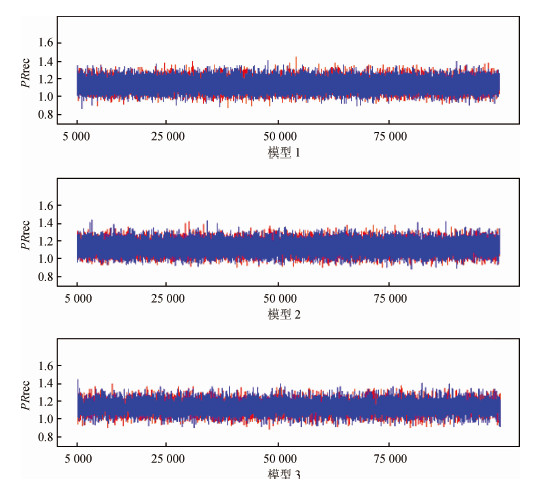图 1 贝叶斯log-binomial回归模型看护人识别腹泻危险症状变量的迭代历史轨迹图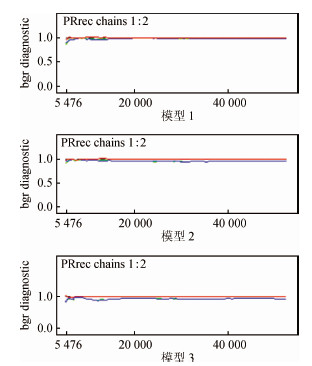图 2 贝叶斯log-binomial回归模型看护人识别腹泻危险症状变量的迭代历史轨迹图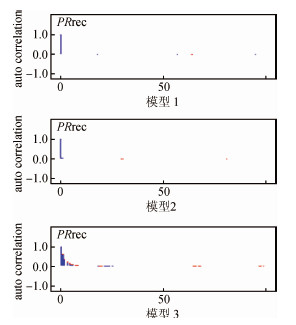图 3 贝叶斯log-binomial模型看护人识别腹泻危险症状变量PR的自相关图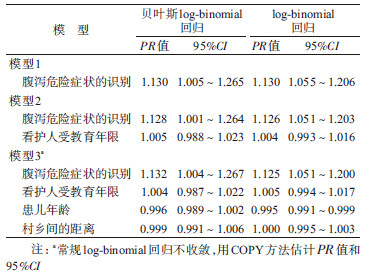Rodriguez-Alvarez E, Borrell LN, González-Rábago Y, et al. Induced abortion in a southern European region:examining inequalities between native and immigrant women[J]. Int J Public Health, 2016, 61(7): 829–836. DOI:10.1007/s00038-016-0799-7  Chang TI, Evans G, Cheung AK, et al. Patterns and correlates of baseline thiazide-type diuretic prescription in the systolic blood pressure intervention trial[J]. Hypertension, 2016, 67(3): 550–555. DOI:10.1161/HYPERTENSIONAHA.115.06851  Magnus MC, KarlstadØ, Håberg SE, et al. Prenatal and infant paracetamol exposure and development of asthma:the Norwegian Mother and Child Cohort study[J]. Int J Epidemiol, 2016, 45(2): 512–522. DOI:10.1093/ije/dyv366  Albert JH, Chib S. Bayesian analysis of binary and polychotomous response data[J]. J Am Statist Assoc, 1993, 88(422): 669–679. DOI:10.1080/01621459.1993.10476321  Kruschke JK.Doing Bayesian data analysis-a tutorial with R and BUGS[M].Burlington, MA: Academic Press, 2010.  Deggens JA, Petersen MR, Lei X. Estimation of prevalence ratios when PROC GENMOD does not converge[C]//Proceedings of the 28th Annual SAS Users Group International Conference. Cary, NC:SAS Institute Inc, 2003:270.  叶荣, 郜艳晖, 杨翌, 等. log-binomial模型估计的患病比及其应用[J]. 中华流行病学杂志, 2010, 31(5): 576–578. Ye R, Gao YH, Yang Y, et al. Using log-binomial model for estimating the prevalence ratio[J]. Chin J Epidemiol, 2010, 31(5): 576–578. DOI:10.3760/cma.j.issn.0254-6450.2010.05.024  Chu HT, Cole SR. Estimation of risk ratios in cohort studies with common outcomes:a Bayesian approach[J]. Epidemiology, 2010, 21(6): 855–862. DOI:10.1097/EDE.0b013e3181f2012b  Spiegelhalter D, Thomas A, Best N, et al. Open BUGS user manual (version 3.2.3)[M]. Cambridge:MRC Biostatistics Unit, 2014.  Lee J, Chia KS. Estimation of prevalence rate ratios for cross sectional data:an example in occupational epidemiology[J]. Br J Ind Med, 1993, 50(9): 861–862.  Savu A, Liu Q, Yasui Y. Estimation of relative risk and prevalence ratio[J]. Stat Med, 2010, 29(22): 2269–2281. DOI:10.1002/sim.3989  Zou GY. A modified Poisson regression approach to prospective studies with binary data[J]. Am J Epidemiol, 2004, 159(7): 702–706. DOI:10.1093/aje/kwh090  Yelland LN, Salter AB, Ryan P. Performance of the modified Poisson regression approach for estimating relative risks from clustered prospective data[J]. Am J Epidemiol, 2011, 174(8): 984–992. DOI:10.1093/aje/kwr183  McNutt LA, Wu CT, Xue XN, et al. Estimating the relative risk in cohort studies and clinical trials of common outcomes[J]. Am J Epidemiol, 2003, 157(10): 940–943. DOI:10.1093/aje/kwg074  Skove T, Deddens J, Petersen MR, et al. Prevalence proportion ratios:estimation and hypothesis testing[J]. Int J Epidemiol, 1998, 27(1): 91–95. DOI:10.1093/ije/27.1.91  Zhang J, Yu KF. What's the relative risk? A method of correcting the odds ratio in cohort studies of common outcomes[J]. JAMA, 1998, 280(19): 1690–1691. DOI:10.1001/jama.280.19.1690  Schouten EG, Dekker JM, Kok FJ, et al. Risk ratio and rate ratio estimation in case-cohort designs:hypertension and cardiovascular mortality[J]. Stat Med, 1993, 12(18): 1733–1745. DOI:10.1002/sim.4780121808